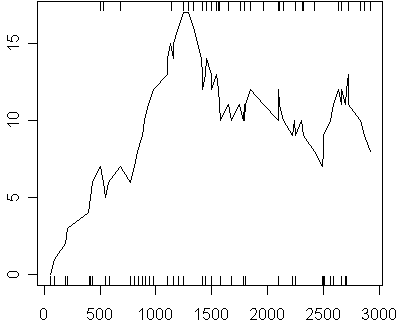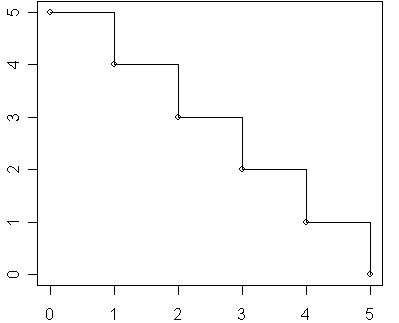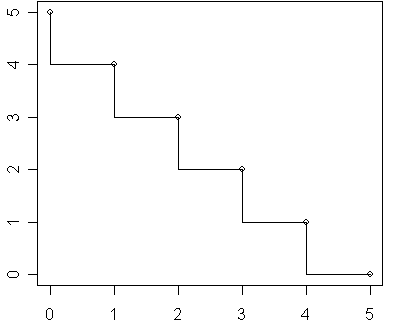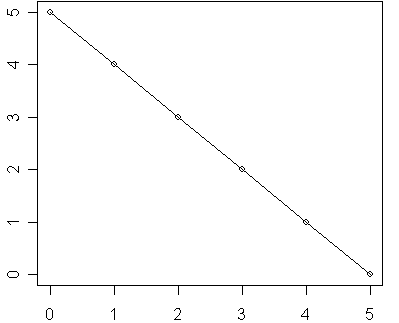# Stair step interpolation in R

## 2007-11-15

I am working on some charts that show discrete (sudden) jumps at specific time points. This requires the use of stair step interpolation, because if you just connected the lines, it would imply 1a linear transition between consecutive points. Here’s an example of the plot I want.It shows the number of patients at risk during any time point in the study. The number at risk has to be a whole number because it is impossible to have half a patient at risk in a study.

Here’s what it would look like if I just connected the dots.The programming language R allows you to connect the points either a linear transition (which is what you want for most plots) or using a stair step transition. With a stair step transition, you have the choice of moving first horizontally and then vertically, or vice versa. If you specify a lower case s for the type of graph, R will move horizontally first during the transition. If you specify an upper case S, R will move vertically first during the transition. If you specify a lower case l, R will use a linear transition between data points.

Here’s a simple example. The following R code

plot(0:5,5:0)
lines(0:5,5:0,type="s")


will produce this plot.Notice that when you move from (5,0) to (4,1), you produce horizontally along y=5 and then drop down to 4 when x reaches 1. Change the R code to this

plot(0:5,5:0)
lines(0:5,5:0,type="S")


and you get this plot instead.Now when you move from (5,0) to (4,1), you drop veritcally to 4 first and then proceed horizontally until you reach x=1. It a subtle difference, but it is important. Finally, use this code

plot(0:5,5:0)
lines(0:5,5:0,type="l")


to get this plotThis plot shows a linear transition between (5,0) and (4,1).

You can find an earlier version of this page on my old website.